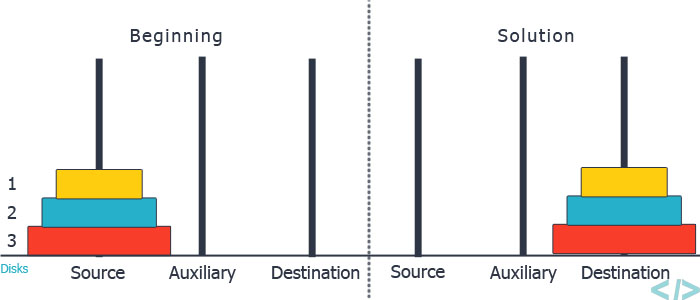# Tower of Hanoi

Posted: 19 Dec, 2020
Difficulty: Easy

## PROBLEM STATEMENT

#### You are given three rods (numbered 1 to 3), and ‘N’ disks initially placed on the first rod, one on top of each other in increasing order of size ( the largest disk is at the bottom). You are supposed to move the ‘N’ disks to another rod(either rod 2 or rod 3) using the following rules and in less than 2 ^ (N) moves.

``````1. You can only move one disk in one move.
2. You can not place a larger disk on top of a smaller disk.
3. You can only move the disk at the top of any rod.
``````
##### Note :
``````You may assume that initially, the size of the ‘i’th disk from the top of the stack is equal to ‘i’, i.e. the disk at the bottom has size ‘N’, the disk above that has size ‘N - 1’, and so on. The disk at the top has size 1.
``````
##### Example :##### Input Format :
``````The first line contains a single integer ‘T’ denoting the number of test cases. The test cases follow.

The first line of each test case contains a single integer ‘N’ denoting the number disks.
``````
##### Output Format :
``````For each test case, return a 2-D array/list, where each row of the array should contain exactly two integers. The first integer should be the number of the rod from where the topmost disk is to be removed and the second integer should denote the number of the rod where the removed disk is to be placed. If you have correctly moved all the disks from rod 1 to either rod 2 or rod 3 the output will be ‘1’ otherwise the output will be ‘0’.

The output of every test case will be printed in a separate line.
``````
##### Note :
``````You don’t need to print anything; It has already been taken care of.
``````
##### Constraints :
``````1 <= T <= 5
1 <= N <= 12

Where ‘T’ denotes the number of test cases, ‘N’ denotes the number of disks.

Time Limit: 1 sec
``````Approach 1

The idea is to use recursion to solve the problem. Firstly, we will try to solve this problem for N = 2; we can move a disk from rod 1 to rod 2, then move another disk from rod 1 to rod 2, and then move the disk in rod 2 to rod 3, this way we can move all the disks to rod 3. Now, to solve for N = 3, we can first solve the problem for the first two disks and then move the last disk to another rod and then place the two disks on top of the last disk.

Generalizing this approach, to solve the problem for ‘N’ disks we will first solve the problem for ‘N-1’ disks and then move the last disk to another rod, thus completely solving the problem for ‘N’ disks.

The steps are as follows :

1. Let’s define a recursive function as moveDisk(n, toRod, fromRod, moves). Here, ‘n’ denotes the number of disks we are solving the problem for, “toRod” denotes the number of the rod to which we will move the disk to, “fromRod” denotes the number of the rod from where we will remove the disk and “moves” is a 2-D array for storing the moves that we will perform.
1. If the value of ‘n’ is 1 then simply move the only disk from “fromRod” to “toRod” and add the values of “to” and “from” in the moves array.
2. Otherwise, find the number of the remaining rod (rod other than “toRod” and “fromRod”), let’s say this rod is “rem”. Now recursively move the top ‘n - 1’ disks from “fromRod” to “rem” rod by calling moveDisk(n - 1, rem, fromRod, moves).
3. Now we have removed the top ‘n - 1’ disks from “fromRod” and placed them on ”rem” rod; hence we will move the last disk (n’th disk) of the “fromRod” to the “toRod” and add the values of “toRod” and “fromRod” in the answer.
4. After this, we need to move the remaining n - 1 disks from the “rem” rod to “toRod”, thus we will again recursively call the function, moveDisk(n - 1, ”toRod”, “rem”, moves).
2. Now we have moved all the ‘N’ disks to another rod; hence we will return the moves array.